###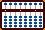WHERE TO PLACE QUOTIENT ANSWERS

When it comes to solving problems of long division on the soroban, I find that I can become confused when quotient answers contain a lot of zeros. Sometimes it can be difficult to sort out which quotient numbers should be placed on which rods. The following is a good way to keep track. This technique works so well, placing quotient answers on the soroban becomes virtually effortless. In fact the method is so effective, you may find you'll never be wrong again. :)

Before tackling this, it's a good idea to understand Takashi Kojima's method for solving problems of long division.

#### Example: 927 ? 3

Rule 1) If the digits in the divisor are less than (or equal to) the corresponding digits of the dividend, place the first quotient number two rods to the left of the dividend. Note that in Fig. A, the divisor 3 is smaller than the dividend 9. Therefore the quotient number is placed two rods to the left of the dividend.

Fig. A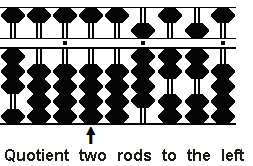#### Example: 273 ? 3

Rule 2) If the digits in the divisor are greater than the corresponding digits of the dividend, place the first quotient number one rod to the left of the dividend. Note that in Fig. B, the divisor 3 is larger than the dividend 2. Therefore the quotient number is placed one rod to the left of the dividend.

Fig. B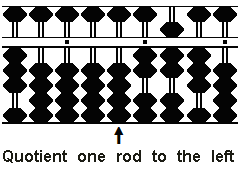In the following example I've chosen rod E to be the unit rod and set up the problem on the soroban following using techniques for predetermining the unit rod. This way the unit number in the quotient will naturally fall on unit rod E with the first decimal falling on rod F. Using the Professor's method, what looks to be a complicated decimal problem becomes much more manageable. All we have to do is set 3908 ? 39 onto the frame knowing the soroban will naturally produce the correct decimal answer.

 ******* Please don't let the long quotient answer fool you into thinking this is too complicated a problem to do on the soroban. In fact, it's using a soroban that makes it all so easy. *******

Example:  3.908 ? 0.39 = 10.0205128

Step 1: Rod E will be the unit rod. Using Professor Kato's method, set the dividend 3908 on rods FGHI and the divisor 39 on rods AB. (Fig.1)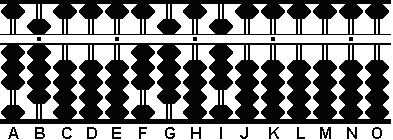(Fig.1) ```Step 1 A B C D E F G H I J K L M N O . . . .   .   3 9 0 0 0 3 9 0 8 0 0 0 0 0 0 Step 1 ```

Because the divisor 39 on rods on AB is equal to the 39 on FG, apply "Rule 1" and begin by placing the quotient two rods to the left of the dividend on rod D.

Step 2: Divide 39 on rods AB into 39 on rods FG  and place the quotient 1 on rod D.

2a: Multiply the 1 on D by the 39 on AB and subtract the product 39 from 39 on rods FG, leaving the partial product 1 on rod D and the remainder of the dividend 8 on rod I. (Fig.2)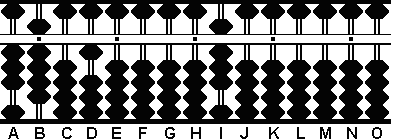(Fig.2) ```Step 2 A B C D E F G H I J K L M N O . . . .   .   3 9 0 0 0 3 9 0 8 0 0 0 0 0 0 (1) Step 2 - 3 9 Step 2a 3 9 0 1 0 0 0 0 8 0 0 0 0 0 0 ```

Note: We'll continue to solve this problem as far as the soroban will allow by borrowing zeros from rods on the right.

This is where it might look complicated but following the rules makes it easy. With 1 on rod D and 8 on rod I (see Fig.2), there are 4 empty rods in between. Where to place the next quotient number? This might be confusing. But if we follow the rules there's an easy solution. Because the divisor 3 on rod A is smaller than the dividend 8 on rod I, follow "Rule 1" and place the next quotient number two rods to the left of the dividend. This takes us to rod G. It's that simple.

Step 3: Divide 3 on A into 8 on rod I and place the quotient 2 on rod G.

3a: Multiply the quotient 2 on G by the 3 on A and subtract the product 6 from 8 on rod I.

3b: Multiply the quotient 2 on G by the 9 on B and subtract the product 18 from rods IJ. This leaves the partial quotient 1002 on rods DEFG and the remainder 2 on rod J. (Fig.3)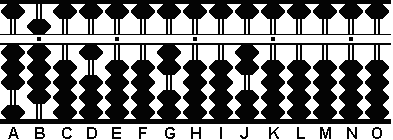(Fig.3) ```Step 3 A B C D E F G H I J K L M N O . . . .   .   3 9 0 1 0 0 0 0 8 0 0 0 0 0 0 (2) Step 3 - 6 Step 3a 3 9 0 1 0 0 2 0 2 0 0 0 0 0 0 - 1 8 Step 3b 3 9 0 1 0 0 2 0 0 2 0 0 0 0 0 ```

With rods H & I both empty (see Fig.3), once again we're there's a decision to be made. Where to place the next quotient number? Because the 3 on A is larger than the 2 on rod J, Follow "Rule 2" and place the next quotient number one rod to the left of the dividend on rod I.

Step 4: Divide 3 on A into 20 on rods JK and place the quotient 5 on rod I.

4a: Multiply the quotient 5 on rod I by the 3 on A and subtract the product 15 from 20 on rods JK.

4b: Multiply the quotient 5 by the 9 on B and subtract the product 45 from rods KL. This leaves the partial quotient 100205 on rods DEFGHI and the remainder 5 on rod L. (Fig.4)(Fig.4) ```Step 4 A B C D E F G H I J K L M N O . . . .   .   3 9 0 1 0 0 2 0 0 2 0 0 0 0 0 (5) Step 4 - 1 5 Step 4a 3 9 0 1 0 0 2 0 5 0 5 0 0 0 0 - 4 5 Step 4b 3 9 0 1 0 0 2 0 5 0 0 5 0 0 0 ```

With rods J & K both empty (see Fig.4), once again we're faced with a decision. Where to place the next quotient number? Because the 3 on A is smaller than the 5 on rod L, Follow "Rule 1" and place the next quotient number two rods to the left of the dividend on rod J.

Step 5: Divide 3 on A into 5 on rod L and place the quotient 1 on rod J.

5a: Multiply the quotient 1 on rod J by the 3 on A and subtract the product 3 from 5 on rod L.

5b: Multiply the quotient 1 by the 9 on B and subtract the product 9 from rod M. This leaves the partial quotient 1002051 on rods DEFGHIJ and the remainder 11 on rods LM. (Fig.5)(Fig.5) ```Step 5 A B C D E F G H I J K L M N O . . . .   .   3 9 0 1 0 0 2 0 5 0 0 5 0 0 0 (1) Step 5 - 3 Step 5a 3 9 0 1 0 0 2 0 5 1 0 2 0 0 0 - 9 Step 5b 3 9 0 1 0 0 2 0 5 1 0 1 1 0 0 ```

Follow "Rule 2" and place the next quotient number right next to the dividend on rod K.

Step 6: Divide 3 on A into 11 on rods LM and place the quotient 2 on rod K.

6a: Multiply the quotient 2 on rod J by the 3 on A and subtract the product 6 from 11 on rods LM.

6b: Multiply the quotient 2 by the 9 on B and subtract the product 18 from rods MN. This leaves the partial quotient 10020512 on rods DEFGHIJK and the remainder 32 on rods MN. (Fig.6)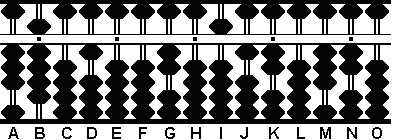(Fig.6) ```Step 6 A B C D E F G H I J K L M N O . . . .   .   3 9 0 1 0 0 2 0 5 1 0 1 1 0 0 (2) Step 6 - 6 Step 6a 3 9 0 1 0 0 2 0 5 1 2 0 5 0 0 - 1 8 Step 6b 3 9 0 1 0 0 2 0 5 1 2 0 3 2 0 ```

Here the 39 on rods AB is greater than the 32 on rods MN (see Fig.6). Follow "Rule 2" and place the next quotient number right next to the dividend on rod L.

Step 7: Divide 3 on A into 32 on rods MN and place the quotient 8 on rod L.

7a: Multiply the quotient 8 on rod L by the 3 on A and subtract the product 24 from 32 on rods MN.

7b: Multiply the quotient 8 by the 9 on B and subtract the product 72 from rods NO. This leaves the partial quotient 100205128 on rods DEFGHIJKL and the remainder 8 on rod O. (Fig.6).(Fig.7) ```Step 7 A B C D E F G H I J K L M N O . . . .   .   3 9 0 1 0 0 2 0 5 1 2 0 3 2 0 (8) Step 7 -2 4 Step 7a 3 9 0 1 0 0 2 0 5 1 2 0 0 8 0 - 7 2 Step 7b 3 9 0 1 0 0 2 0 5 1 2 8 0 0 8 ```

Conclusion: This is as far as this soroban will let us go. Disregarding the last three rods and taking into account that rod E was designated as the unit rod, the answer on the soroban reads 10.0205128. Not bad for less than a minutes work, eh? :)

REFERENCES:

Kato, Professor Fukutaro.
SOROBAN pelo M?todo Moderno (SOROBAN by the Modern Method)
Brazil: Brazilian Shuzan Cultural Association, (not dated ? but looks to be circa 1969)

Kojima, Takashi.
The Japanese Abacus: Its Use and Theory
Tokyo: Charles E. Tuttle, 1954

Martinez, Beluva Sulliuent.
Soroban in America
Tokyo: The league of Soroban Education of Japan Inc. (not dated - but looks to be circa 1980)

Print Page (.pdf format,164kb)